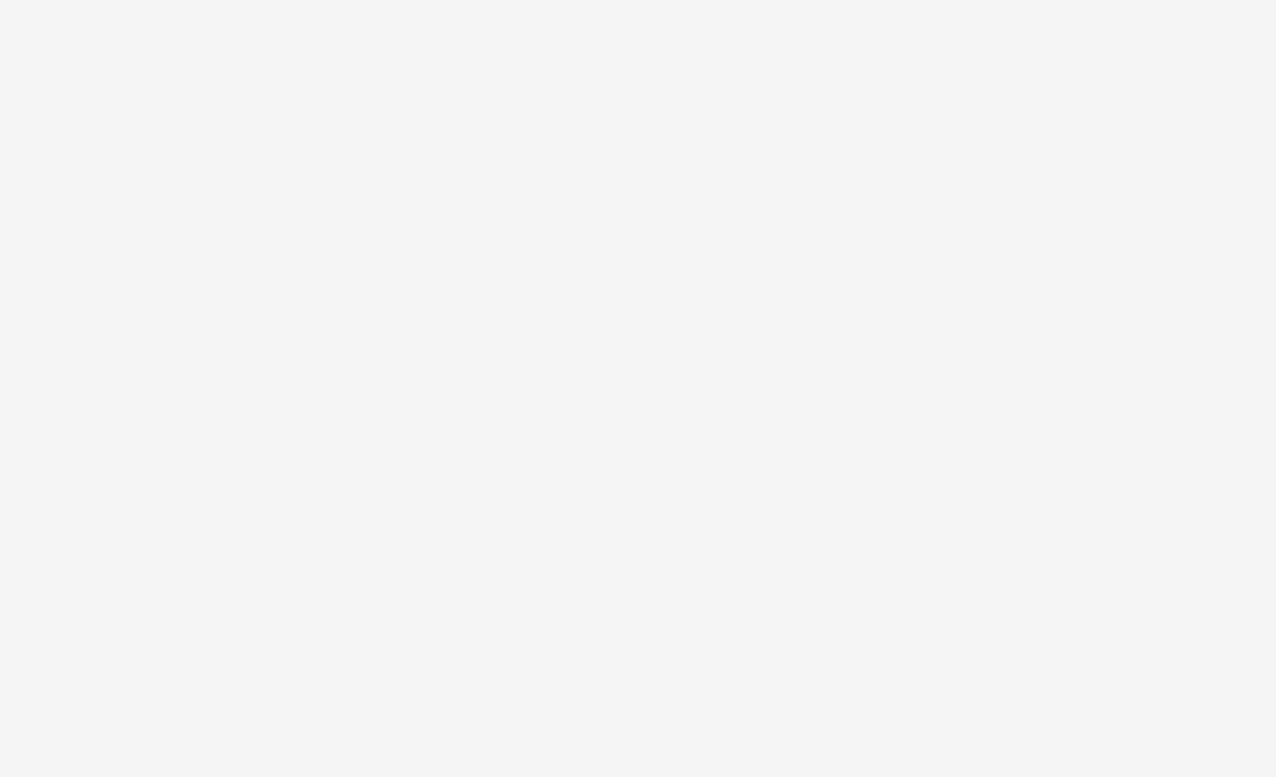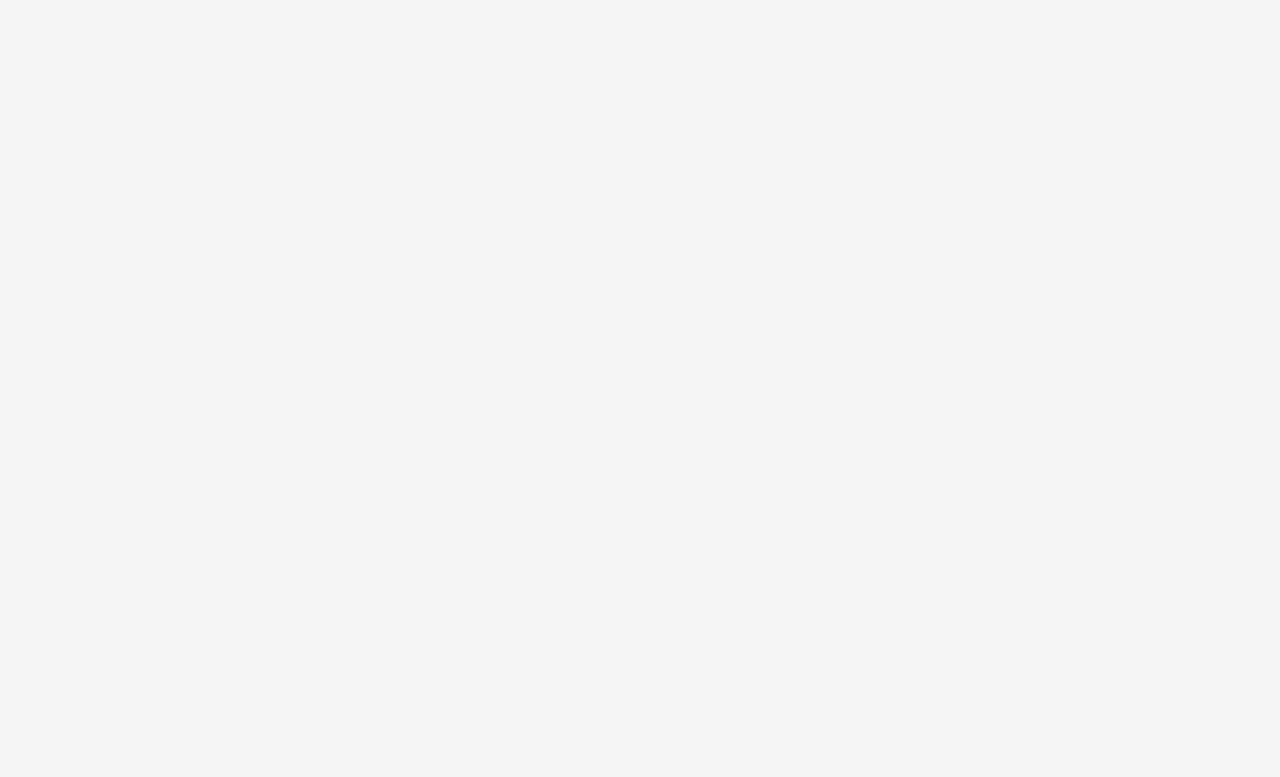How to calculate the age based on date of birth entered by the user?

# How to calculate the age based on date of birth entered by the user?

### You can use the DIFF function to calculate the age based on the date of birth entered by the user.#### Instructions to calculate age#### Use DIFF and TODAY function to calculate age

Diff function calculates the number of seconds, minutes, hours, days, months or years between two date or date & time questions. Since we want to calculate the age, we will use years as the unit.

TODAY().diff(date_of_birth, "years")

Note: To enter date_of_birth, type @ in the calculate option and select the date of birth question from the list.